# 从头开始学习梯度下降#### 目录

• 什么是梯度下降，为什么它很重要？

• 梯度下降背后的直觉

• 梯度下降背后的数学

• 具有 1 个变量的梯度下降代码

• 具有 2 个变量的梯度下降

• 学习率的影响

• 损失函数的影响

• 数据效果

• 尾注

#### 梯度下降背后的直觉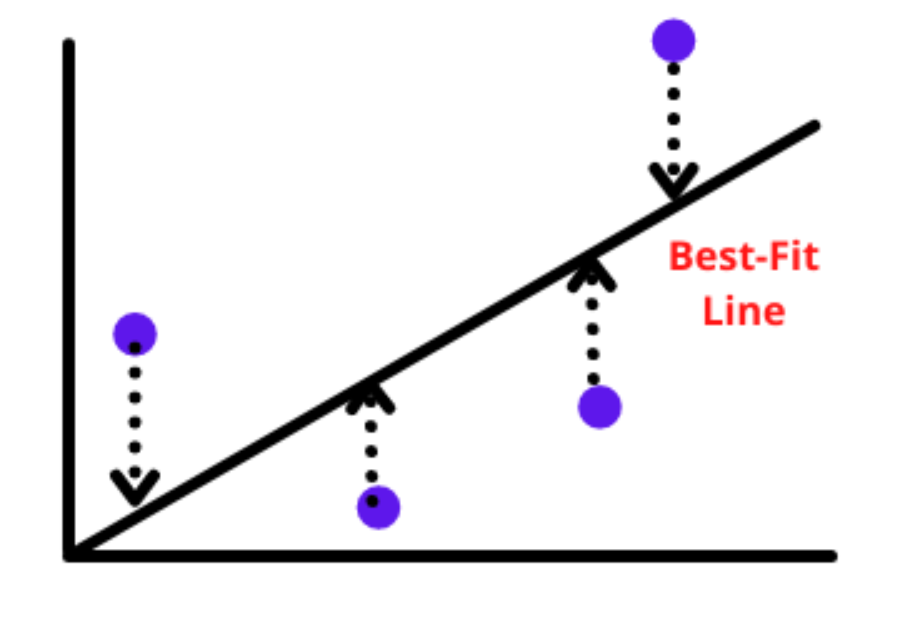我们必须找到最佳拟合直线，当损失最小时，给出b的最小值。损失函数定义为实际值和预测值之间的差的平方和。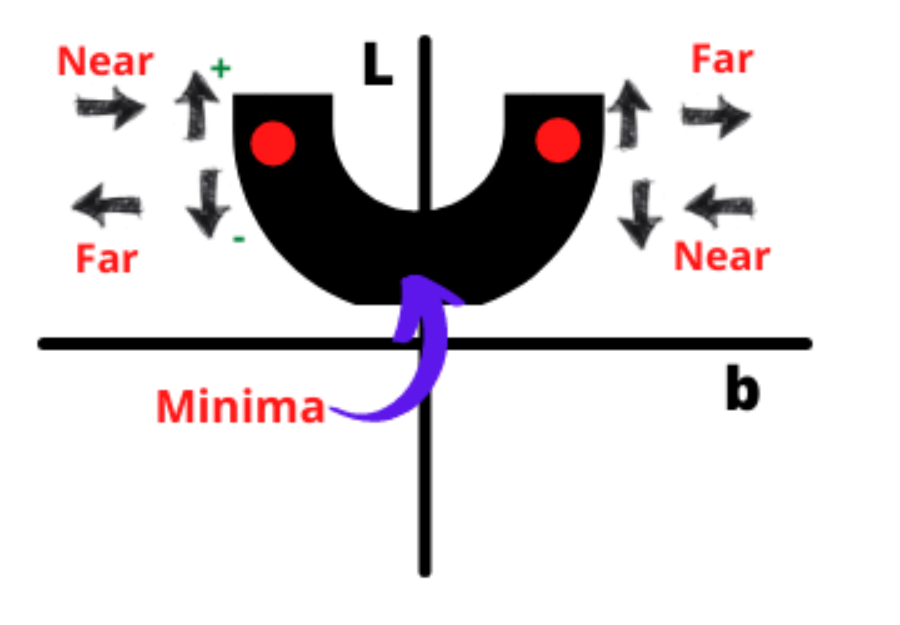b_new = b_old - slope



b_new = b_old - learning rate * slope



1. 当 b_new – b_old = 0 表示我们没有前进，所以我们可以停下来。

2. 我们可以将迭代次数限制为 1000 次。多次迭代称为 epoch，我们可以将其初始化为超参数。

#### 梯度下降背后的数学

for i in epochs:
b_new = b_old - learning_rate * slope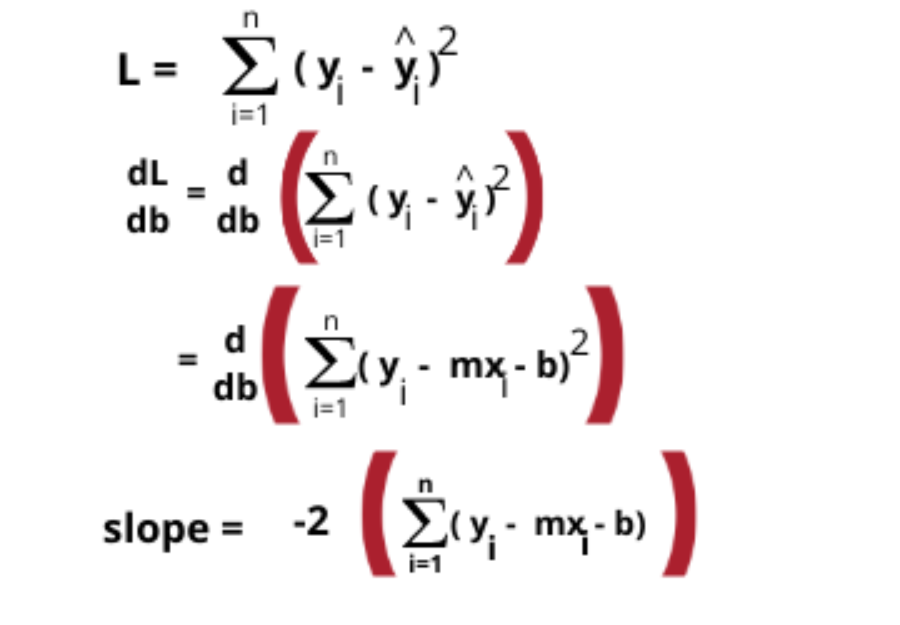#### 具有1个变量的梯度下降代码

from sklearn.datasets import make_regression
import numpy as np
import matplotlib.pyplot as plt
X,y = make_regression(n_samples=4, n_features=1, n_informative=1, n_targets=1, noise=80, random_state=13)
plt.scatter(X,y)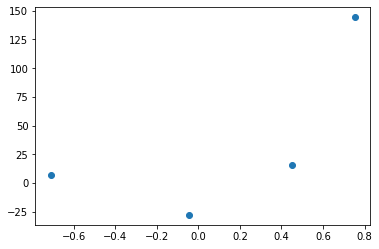from sklearn.linear_model import LinearRegression
reg = LinearRegression()
reg.fit(X,y)
print(reg.coef_)
print(reg.intercept_)



plt.scatter(X,y)
plt.plot(X,reg.predict(X),color='red')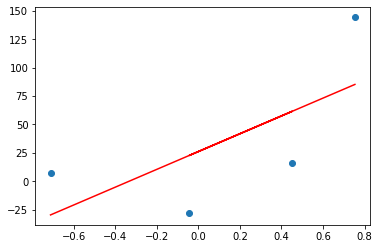y_pred = ((78.35 * X) + 0).reshape(4)
plt.scatter(X,y)
plt.plot(X,reg.predict(X),color='red',label='OLS')
plt.plot(X,y_pred,color='#00a65a',label='b = 0')
plt.legend()
plt.show()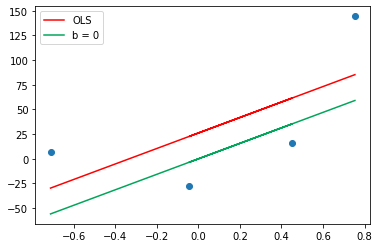m = 78.35
b = 0
loss_slope = -2 * np.sum(y - m*X.ravel() - b)
# Lets take learning rate = 0.1
lr = 0.1
step_size = loss_slope*lr
print(step_size)
# Calculating the new intercept
b = b - step_size
print(b)



loss_slope = -2 * np.sum(y - m*X.ravel() - b)
step_size = loss_slope*lr
b = b - step_size
print(b)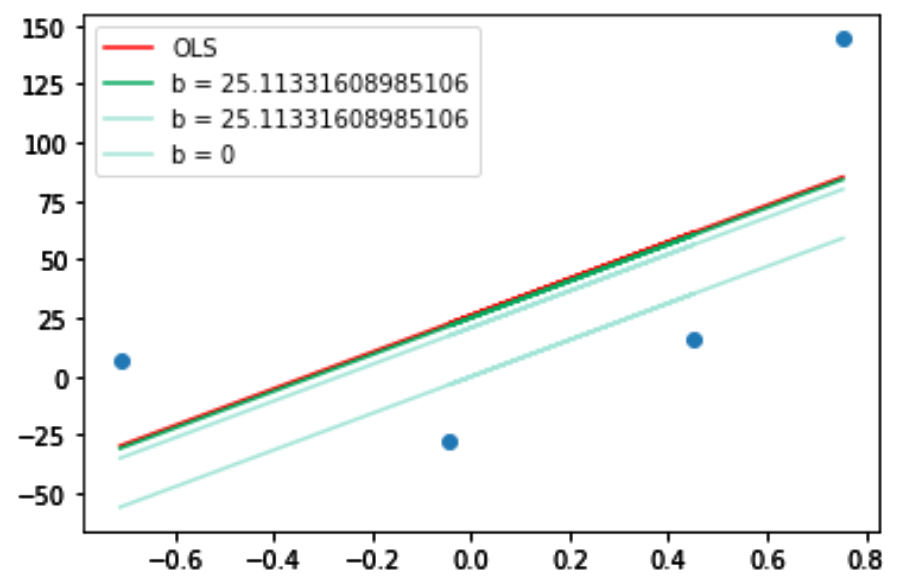#### 2个变量的梯度下降

Step-1) 初始化m和b的随机值

Step-2）初始化epoch数和学习率

Step-3) 在迭代中开始计算斜率和截距

for i in epochs:
b_new = b_old - learning_rate * slope
m_new = m_old - learning_rate * slope



from sklearn.datasets import make_regression
X, y = make_regression(n_samples=100, n_features=1, n_informative=1, n_targets=1, noise=20, random_state=13)



class GDRegressor:
def __init__(self, learning_rate, epochs):
self.m = 100
self.b = -120
self.lr = learning_rate
self.epochs = epochs
def fit(self, X, y):
#calculate b and m using GD
for i in range(self.epochs):
loss_slope_b = -2 * np.sum(y - self.m * X.ravel() - self.b)
loss_slope_m = -2 * np.sum((y - self.m * X.ravel() - self.b)*X.ravel())
self.b = self.b - (self.lr * loss_slope_b)
self.m = self.m - (self.lr * loss_slope_m)
print(self.m, self.b)
def predict(self, X):
return self.m * X + self.b
#create object and check algorithm
gd = GDRegressor(0.001, 50)
gd.fit(X, y)



#### 尾注

☆ END ☆09-19128
09-21122
09-22134
05-021万+
09-18149
03-261200
09-2280
09-20109
09-19182
09-2088
09-16209
09-1998
09-19135
09-20139

### “相关推荐”对你有帮助么？

•非常没帮助
•没帮助
•一般
•有帮助
•非常有帮助被折叠的  条评论 为什么被折叠?到【灌水乐园】发言woshicver

¥1 ¥2 ¥4 ¥6 ¥10 ¥20获取中扫码支付点击重新获取扫码支付1.余额是钱包充值的虚拟货币，按照1:1的比例进行支付金额的抵扣。
2.余额无法直接购买下载，可以购买VIP、付费专栏及课程。余额充值